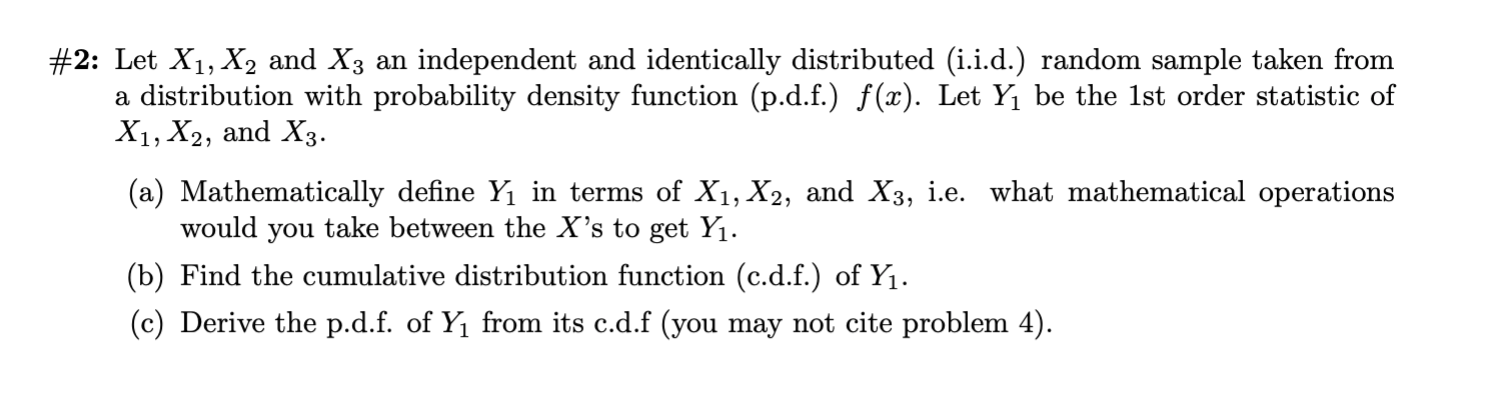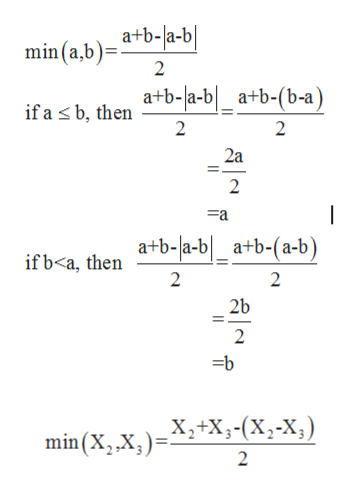# #2: Let X1, X2 and X3 an independent and identically distributed (i.i.d.) random sample taken froma distribution with probability density function (p.d.f.) f(x). Let Y1 be the 1st order statistic ofX1, X2, and X3(a) Mathematically define Yı in terms of X1, X2, and X3, i.e. what mathematical operationswould you take between the X's to get Yı.(b) Find the cumulative distribution function (c.d.f.) of Y1(c) Derive the p.d.f. of Y1 from its c.d.f (you may not cite problem 4)

Question
303 viewshelp_outlineImage Transcriptionclose#2: Let X1, X2 and X3 an independent and identically distributed (i.i.d.) random sample taken from a distribution with probability density function (p.d.f.) f(x). Let Y1 be the 1st order statistic of X1, X2, and X3 (a) Mathematically define Yı in terms of X1, X2, and X3, i.e. what mathematical operations would you take between the X's to get Yı. (b) Find the cumulative distribution function (c.d.f.) of Y1 (c) Derive the p.d.f. of Y1 from its c.d.f (you may not cite problem 4) fullscreen
check_circle

Step 1

Let X1, X2 and X3 an independent and identically distributed (i.i.d) random sample taken from a distribution with probability density function (p.d.f) f(x).

Step 2

(a) Let Y1 be the 1st order statistic of X1, X2 and X3.

Step 3

We know th...help_outlineImage Transcriptionclosea+b-la-b min (a,b) 2 a+b-la-b_a+b-(b-a) if a s b, then 2 2 2a 2 -a a+b-la-b_a+b-(a-b) if b

### Want to see the full answer?

See Solution

#### Want to see this answer and more?

Solutions are written by subject experts who are available 24/7. Questions are typically answered within 1 hour.*

See Solution
*Response times may vary by subject and question.
Tagged in

### Statistics